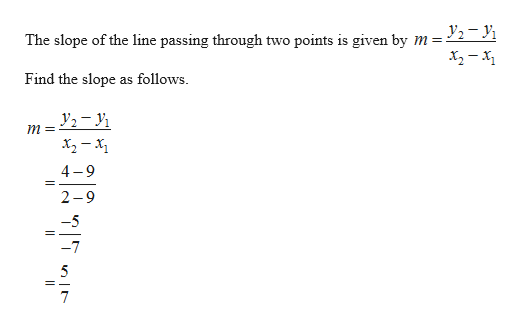# Find the slope m of the line containing the points (9, 9) and (2, 4). (Enter an exact number as an integer, fraction, or decimal. If an answer is undefined, enter UNDEFINED.)m =

Question
7 views

Find the slope m of the line containing the points (9, 9) and (2, 4). (Enter an exact number as an integer, fraction, or decimal. If an answer is undefined, enter UNDEFINED.)
m =

check_circle

Step 1

The given points are (9,9) an...help_outlineImage Transcriptionclosey2- The slope of the line passing through two points is given by m = Find the slope as follows. m= 4 9 2-9 -5 7 fullscreen

### Want to see the full answer?

See Solution

#### Want to see this answer and more?

Solutions are written by subject experts who are available 24/7. Questions are typically answered within 1 hour.*

See Solution
*Response times may vary by subject and question.
Tagged in

### Other